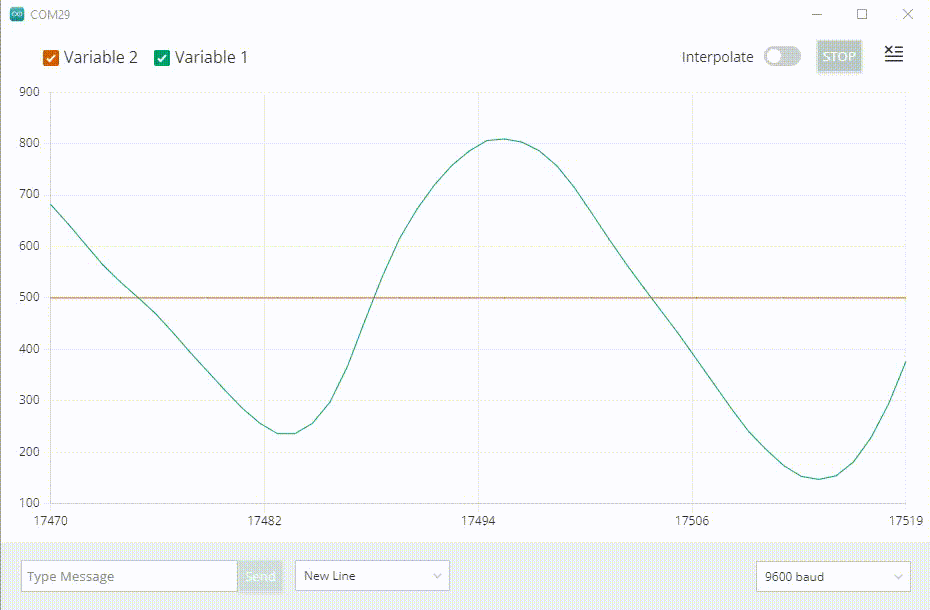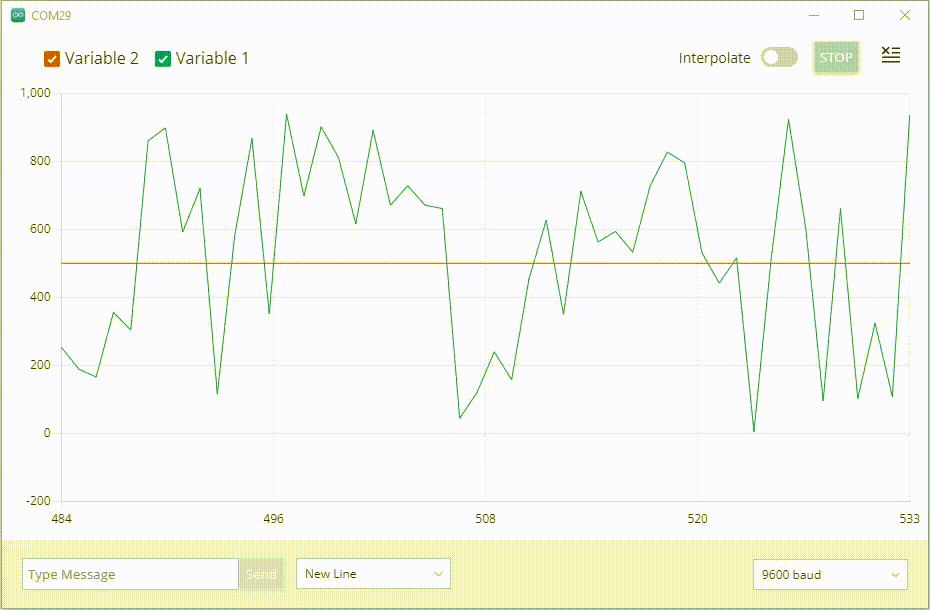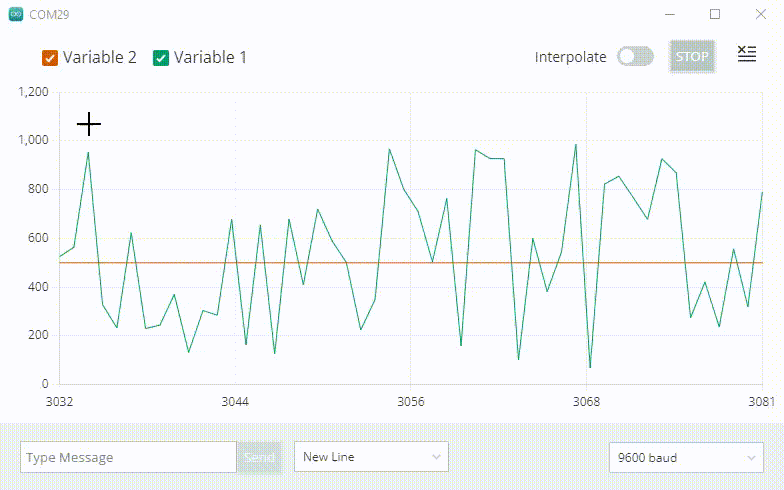# Using the Serial Plotter Tool

Learn how to setup and use the Serial Plotter in the Arduino IDE 2.

The Serial Plotter tool is a versatile tool for tracking different data that is sent from your Arduino board. It functions similarly to your standard Serial Monitor tool which is used to print data "terminal style", but is a greater visual tool that will help you understand and compare your data better.

In this tutorial, we will take a quick look on how to enable this feature (works for practically any sketch that uses serial communication), how a sample sketch looks like, and how it is expected to work.

If you need help to download and install the Arduino IDE 2, you can visit the IDE 2 downloading and installing guide. For other guides on how to use the editor, visit the IDE 2 docs.

## Goals

The goal with this tutorial is:

• Learn how to use the Serial Plotter.
• Create a simple sketch and test it out.

## Example Sketch

To use the Serial Plotter, we will need to create a sketch and upload it to our board. This sketch needs to include at least one numerical variable, such as an

``int``
or
``float``
.

Below you will find two sketches, one using a potentiometer and

``analogRead()``
function, the other using the
``random()``
function. Both sketches have a variable named
``static_variable``
which has a permanent value of
``500``
, used as a reference value.

### Sketch (With Potentiometer)

• ``int potentiometer``
- variable to store value from a potentiometer, connected to an analog pin (gives a value between 0-1023).
• ``int static_variable = 500``
- variable that has an unchanged value of 500.
``````1int potentiometer;2int static_variable = 500;3
4void setup() {5  Serial.begin(9600);6}7
8void loop() {9  potentiometer = analogRead(A1);10
11  Serial.print("Variable_1:");12  Serial.print(potentiometer);13  Serial.print(",");14  Serial.print("Variable_2:");15  Serial.println(static_variable);16}``````

### Sketch (Without Potentiometer)

• ``int random_variable``
- variable that stores a randomized value between 0-1000.
• ``int static_variable = 500``
- variable that has an unchanged value of 500.
``````1int random_variable;2int static_variable = 500;3
4void setup() {5  Serial.begin(9600);6}7
8void loop() {9  random_variable = random(0, 1000);10
11  Serial.print("Variable_1:");12  Serial.print(random_variable);13  Serial.print(",");14  Serial.print("Variable_2:");15  Serial.println(static_variable);16}``````

The Serial Plotter recognizes only CRLF

``(\r\n)``
& LF
``(\n)``
as linebreak characters. So ensure that the either there is a linebreak character after the last variable. You can use
``Serial.print("\n")``
or
``Serial.print("\r\n")``
to introduce a linebreak character at the end. Conversely,
``Serial.println()``
introduces a CRLF character automatically. Further, you can also use
``\t``
(tab) or
`` ``
(space) as a delimiter instead of
``,``
(comma) in the above example.

## The Serial Plotter

Once the sketch is uploaded, we can test out the Serial Plotter.

Make sure the sketch has finished uploading before opening the Serial Plotter. You will see the text "upload complete" in the terminal output.

Click the button in the the top right of the IDE window to open the Serial Plotter.

If you choose the potentiometer example sketch, when turning the knob, you should be creating a "wave-like" pattern in the plotter. Here, we can use the

``static_variable``
as a reference, as we know it is always
``500``
. This is an easy example of just testing out an analog component.If you chose the random example sketch, when you open the plotter you will see very random spikes, as the values fluctuate rapidly.You can also enable/disable the variables by checking the box next to the variable name.## Conclusion

The Serial Plotter is a really useful tool for tracking your variables. It can be used for testing and calibrating sensors, comparing values and other similar scenarios.

To learn more about the Arduino IDE 2 features, you can visit the Arduino IDE 2 docs.